# KSEEB SSLC Class 10 Maths Solutions Chapter 13 Statistics Ex 13.4

In this chapter, we provide KSEEB SSLC Class 10 Maths Solutions Solutions Chapter 13 Statistics Ex 13.4 for English medium students, Which will very helpful for every student in their exams. Students can download the latest KSEEB SSLC Class 10 Maths Solutions Solutions Chapter 13 Statistics Ex 13.4 pdf, free KSEEB SSLC Class 10 Maths Solutions Solutions Chapter 13 Statistics Ex 13.4 pdf download. Now you will get step by step solution to each question.

### Karnataka State Syllabus Class 10 Maths SolutionsChapter 13 Statistics Ex 13.4

Question 1.
The following distribution gives the dialy income of 50 workers of a factory.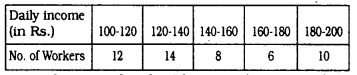Convert the distribution above to a less than type cumulative frequency distribution, and draw its ogive.
Solution: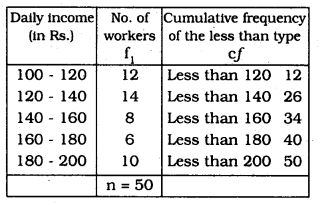n = 50, ∴ n2 = 25
On a graph paper mark the following points :
(120, 12), (140, 26), (160, 34), (180, 40), (200, 50).
For the Ogive graph,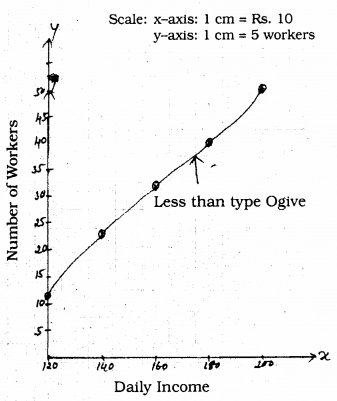Question 2.
During the medical check-up of 35 students of a class, their weights were recorded as follows :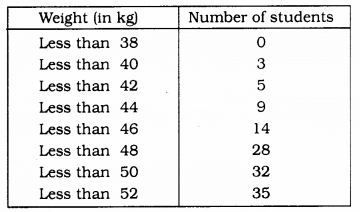Draw a less than type ogive for the given data. Hence obtain the median weight from the graph and verify the result by using the formula.
Solution: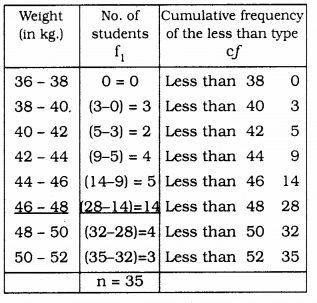To draw ogive of the less than type.
we have to join the points (38, 0), (40, 3), (42, 5), (44, 9), (46, 14), (48, 28), (50, 32), (52, 35).
From the graph, Median is 46.5 kg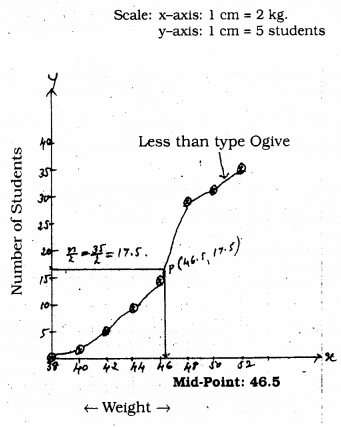n = 35 ∴ n2 = 17.5
Class interval which has median is = (46 – 48)
l = 46, n = 35, f = 14, cf = 14, h = 2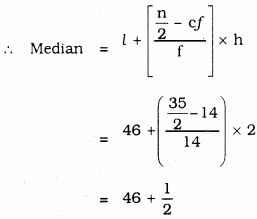= 46.5
∴ Median = 46.5 kg.

Question 3.
The following table gives production yield per hectare of wheat of 100 farms of a village.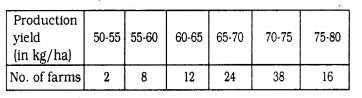Change the distribution to a more than type distribution, and draw its ogive.
Solution: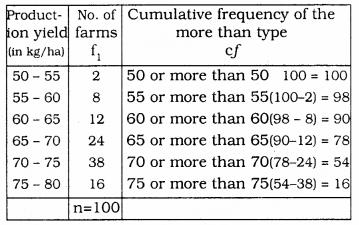We can draw Ogive graph by plotting ordered pairs:
(50, 100), (55, 98), (60, 90), (65, 78). (70, 54), (75, 16).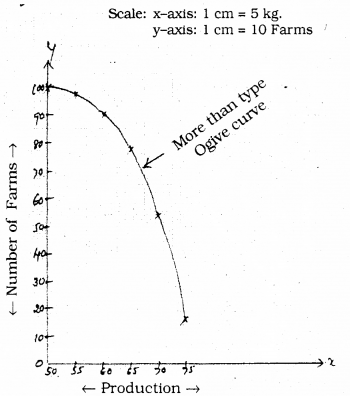All Chapter KSEEB Solutions For Class 10 Maths

—————————————————————————–

All Subject KSEEB Solutions For Class 10

*************************************************

I think you got complete solutions for this chapter. If You have any queries regarding this chapter, please comment on the below section our subject teacher will answer you. We tried our best to give complete solutions so you got good marks in your exam.

If these solutions have helped you, you can also share kseebsolutionsfor.com to your friends.

Best of Luck!!